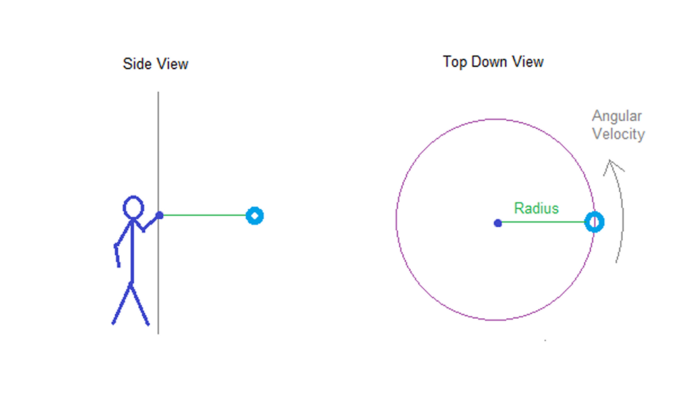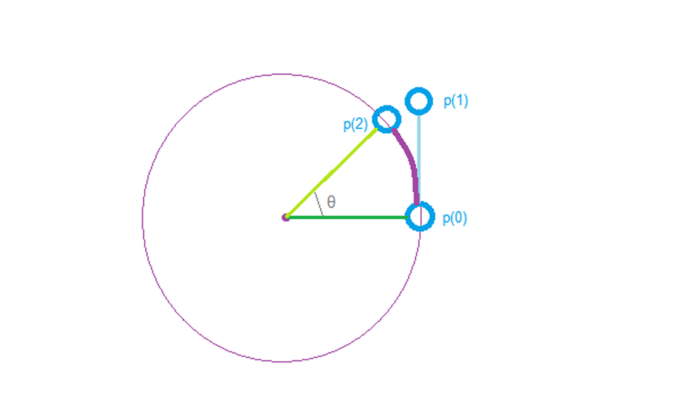# Of Planets and fairground rides

## Musings on objects and motion, part seven, centrifugal “force”

In the last part we covered a little bit about the earth and the moon, we have established there should be a common centre of rotation as both rotate around this common centre. If our understanding of gravity is correct and if our understanding of motion is correct we should be able to work out why the earth and moon haven’t collided?

To go a little bit further, the moon orbits the earth in 27.32 days, thus it takes 2,360,448 seconds to complete one orbit, thus the angular velocity is 8.473×10-7 rad s-1.

Because from observation we know the earth and moon are not colliding there is something preventing this. We know the force acting due to gravity between the bodies is 1.98×1020N. thus both bodies must have an equal and opposite force pulling them outwards.

Because these numbers are large and I struggled to get the moon, let alone the earth as well into my kitchen, lets consider something a bit smaller and familiar. Take a small weight, fix it to a length of string, and (outside, away from windows) swing it around so it describes a circle the centre of which is your hand rotating horizontally around a vertical axis, roughly thus:Now, look at the stone and ask yourself what is going on here and what the stone would like to do (in the horizontal plane)At an initial time the stone is located at p(0) moving with an angular velocity of ω, which is constant, with the known radius r we can work out the actual velocity u, which is variable in direction but fixed in magnitude.

What we need is the length of the arc shown in purple, this combined with the time taken to describe that arc can be used to determine the magnitude of the velocity.

the length of this arc is equal to the angle in radians multiplied by the radius, if we set the angle to be 1 radian and the radius to be 1m we thus can see the arc length is 1m. If we consider this over 1 second with the angular velocity ω = 1 rad s-1 we have a velocity with a magnitude of 1 ms-1.

Consider what would happen without the string (shown in green), the stone would continue to travel in a straight line to the position shown as p1 (again in a horizontal plane, we will ignore the fact it will also fall to the ground for now).

Newton’s laws tell us that unless we provide a force the stone will keep going with a constant velocity, we can observe this effect by letting go of the stone and watching where the stone goes (duck!). After replacing the kitchen window we need to consider why the stone is describing the arc.

What we have is a force acting on the stone provided by the string sufficient to change the velocity such that the final location moves from p1 to p2. This force, towards the centre of the arc being followed by the stone is called centripetal force of “centre seeking” force, this is determined thus:

Fc = mac = mv2 / r

Here v is the magnitude of the velocity (i.e. the speed). If our stone has a mass of 1kg this resolves to centripetal acceleration of 12/1 = 1 ms-2 and a centripetal force of 1N.

So we know the force exerted by the string on the stone, acting towards the centre of the arc, is 1N, this force will cause the stone to describe the arc with the stated angular velocity and mass.

### Centrifugal Force

So what is “centrifugal force”? well this is where it gets interesting. Note the stone is moving under a force provided by the string, the Centripetal Force, as described above, the stone causes a reaction of equal magnitude and opposite direction in the string “pulling” the string outwards, this is the “centrifugal force”, it is however something of a pseudo force, as there is, in practice, no force causing the stone to move away from the centre of the arc being described. This can be seen by cutting the string and the stone will move as described above to p(1). If there was a centrifugal force acting on the stone the stone would move away from the arc.

This force however is real when you consider the string, or anything else fixed by the stone. Consider the classic “water in a bucket” experiment, for the purposes of which we will freeze the water to a block of ice so it acts as a solid. Specifically consider the forces acting. As we have seen the string provides a Centripetal force inwards on the container, the container in turn provides a centrifugal “reaction” force on the string.

But what about the ice? The string is not connected to the ice so cannot provide any force, yet the ice also moves to describe the arc, the container provides the centripetal acceleration to change the velocity of the ice, the ice in turn exerts a reaction acting against this, again the “centrifugal force”.

So, centrifugal force is not really a force as such, it’s the reaction (Newton’s third law) to the centripetal force that basically “balances the equation”, which will vanish the instant the centripetal force is removed.

Ok, back to the earth and the moon, previously we determined the force between these two bodies, which rotate around a common centre. Both experience the same force. As we have seen both are at a different distance from this centre of rotation and their movement describes a circle around it (the moon with a larger radius than the earth).

This force, provided by gravitational attraction, is the “centripetal force” acting upon both bodies. Now if the physics is true we should be able to use the work we have shown to “prove” the orbits are correct, in effect we know the distance (because we have measured it), we know the orbital period (because we can observe it) and we know the force acting on the bodies – if these all add up the maths works.

So lets try it…

Fc = mac = mv2 / r

Consider the moon first

1.98×1020 N = 7.342×1022 kg * v2 / 384,400,000m

we can re-arrange to determine the velocity, which we can then compare to the observed velocity.

v2 = 1.98×1020 N * 384,400,000m / 7.342×1022

this provides a velocity of 1,018.16ms-1

now, what is the observable velocity of the moon?

We determine this based on the fact we know it completes on orbit in 27.3 days, which equates to 2,358,720 seconds. We know the circumference of the orbit is 2,415,256,432m which means we have a velocity of 1,023.97ms-1, now ok these are not exactly the same however given the slight disagreements between various sources of data on the moons orbit (because its not circular for one thing) and various rounding errors is pretty much spot on.

The force of gravity, as determined by the relationship between the mass of the earth and moon, the gravitational constant and the distance between them is pretty much the same as the force required to provide the centripetal acceleration required to keep the moon going around the earth.

This demonstrates two things, firstly why the moon goes around the earth, or more accurately why the earth and the moon go around their common centre of mass. And secondly why the orbit is basically circular.

Note this also works for elliptical orbits (which in practice all orbits are), the proof of this dives into calculus though so will not be handled in detail here. You can however see how it will work from the above – as the orbit increases in distance the centripetal force will reduce as will the angular momentum, these balance out so the object will arc back on its orbit. Newton also observed that for such orbits the area described by the arc in a period of time is constant regardless of which part of the orbit you look at.

In the next part we will consider our first practical question…

© text & diagrams Leopard 2020

The Goodnight Vienna Audio file